Newton's Law Of Motion – MSQ

# Newton's Law Of Motion – MSQ

Test Description

## 10 Questions MCQ Test Topic wise Tests for IIT JAM Physics | Newton's Law Of Motion – MSQ

Newton's Law Of Motion – MSQ for IIT JAM 2022 is part of Topic wise Tests for IIT JAM Physics preparation. The Newton's Law Of Motion – MSQ questions and answers have been prepared according to the IIT JAM exam syllabus.The Newton's Law Of Motion – MSQ MCQs are made for IIT JAM 2022 Exam. Find important definitions, questions, notes, meanings, examples, exercises, MCQs and online tests for Newton's Law Of Motion – MSQ below.
Solutions of Newton's Law Of Motion – MSQ questions in English are available as part of our Topic wise Tests for IIT JAM Physics for IIT JAM & Newton's Law Of Motion – MSQ solutions in Hindi for Topic wise Tests for IIT JAM Physics course. Download more important topics, notes, lectures and mock test series for IIT JAM Exam by signing up for free. Attempt Newton's Law Of Motion – MSQ | 10 questions in 45 minutes | Mock test for IIT JAM preparation | Free important questions MCQ to study Topic wise Tests for IIT JAM Physics for IIT JAM Exam | Download free PDF with solutions
 1 Crore+ students have signed up on EduRev. Have you?
*Multiple options can be correct
Newton's Law Of Motion – MSQ - Question 1

### A painter is applying force himself to raise him and the box with an acceleration of 5 m/s2 by a massless rope and pulley arrangement as shown in figure. Mass of painter is 100kg and that of box is 50kg. If g = 10 m/s2, then :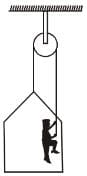Select one or more:

Detailed Solution for Newton's Law Of Motion – MSQ - Question 1

For painter :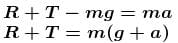.....(1)
For the system;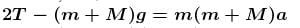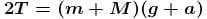..(2)
where; m = 100 kg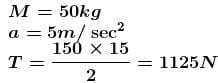and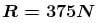The correct answers are: tension in the rope is 1125 N, force of contact between the painter and the floor is 375 N

*Multiple options can be correct
Newton's Law Of Motion – MSQ - Question 2

### Two blocks A and B of equal masses m kg each are connected by a light thread, which passes over a massless pulley as shown in the figure. Both the blocks lies on wedge of mass m kg. Assume friction to be absent everywhere and both the blocks to be always in contact with the wedge. The wedge lying over smooth horizontal surface is pulled towards right with constant acceleration a (m/s2).  (g is acceleration due to gravity)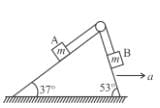Select one or more:

Detailed Solution for Newton's Law Of Motion – MSQ - Question 2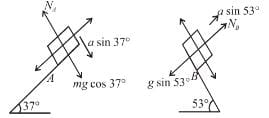The FBD of A and B are
Applying Newton's second law to block A and B along normal to inclined surface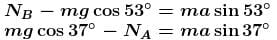Solving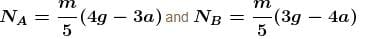For NA to be non zero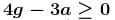or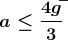The correct answers are:

Normal reaction on A is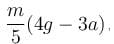Normal reaction on B is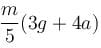Newton's Law Of Motion – MSQ - Question 3

### A light inextensible string connects a block of mass m and top of wedge of mass M. The string is parallel to inclined surface and the inclined surface makes and angle θ with horizontal as show in the figure. All surfaces are smooth. Now a constant horizontal force of minimum magnitude F is applied to wedge towards right such that the normal reaction on block exerted by wedge just becomes zero.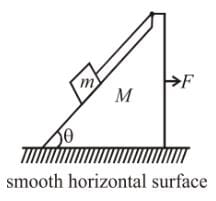Select one or more:

Detailed Solution for Newton's Law Of Motion – MSQ - Question 3

If a is acceleration of wedge, from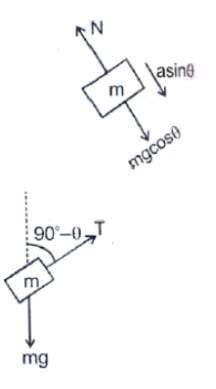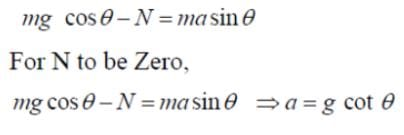*Multiple options can be correct
Newton's Law Of Motion – MSQ - Question 4

Two smooth blocks are placed at a smooth corner as shown in the figure. Both the blocks are having mass m. We apply a force F on the small block m. Block A presses the block B in the normal direction, due to which pressing force on vertical wall will increase, and pressing force on the horizontal wall decrease, as we increase F (θ = 37º  with horizontal). As soon as the pressing force on the horizontal wall by block B becomes zero, it will loose the contact with the ground. If the value of F is further increased, the block B will accelerate in upward direction and simultaneously the block A will move toward right.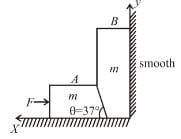Select one or more:

Detailed Solution for Newton's Law Of Motion – MSQ - Question 4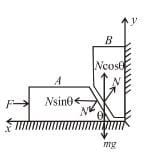For equilibrium of block (A)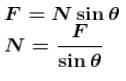To lift block B from ground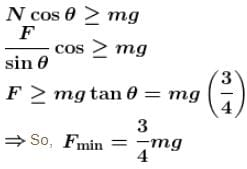To keep regular contact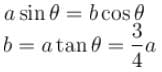The correct answer is: aB = 3a/4

*Multiple options can be correct
Newton's Law Of Motion – MSQ - Question 5

Two blocks of mass M and m, are used to compress two different massless springs as shown in the figure. The left spring is compressed by 3cm, while the right spring is compressed by an unknown amount. The system is at rest and all contact surfaces are smooth. Which of the following statements are true?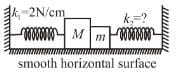Select one or more:

Detailed Solution for Newton's Law Of Motion – MSQ - Question 5

Let F be the force exerted by mass m on mass M.
FBD of mass M
F = k1x1 = 2 × 3 = 6N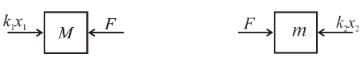FBD of mass m

k2x2 = F = 6N to the left
Hence the force exerted on block of mass m be the right spring (k2x2) is 6 N to the left. From FBD, the normal reaction (F) between blocks is 6 N.
As system is at rest, net force on block of mass m is zero.
The correct answers are: The force exerted on block of mass m by the right spring is 6N to left, The net force on block of mass m is zero, The normal force exerted by block of mass m on block of mass M is 6 N

*Multiple options can be correct
Newton's Law Of Motion – MSQ - Question 6

Two blocks A and B of equal mass m are connected through a massless string and arrangement as shown in the figure. The wedge is fixed on horizontal surface. Friction is absent everywhere. When the system is released from rest.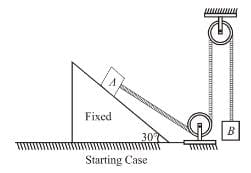Select one or more:

Detailed Solution for Newton's Law Of Motion – MSQ - Question 6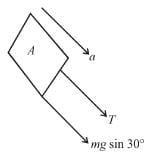Let a be acceleration of system and T be tension in, the string.
F.B.D. of block A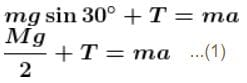F.B.D. of block B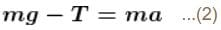Adding equation (1) and (2) we get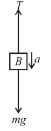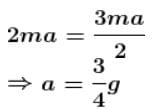from equation (1) ;

T = mg/4
The correct answers are: tension in string is mg/4, acceleration of A is 3g/4

*Multiple options can be correct
Newton's Law Of Motion – MSQ - Question 7

Figure shows two block A and B connected to an ideal pulley string system. In this system when bodies are released then : (neglect friction and take g = 10m/s2)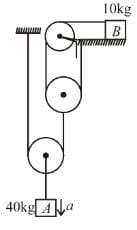Select one or more:

Detailed Solution for Newton's Law Of Motion – MSQ - Question 7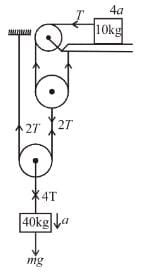Applying NLM on 40kg block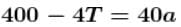For 10kg block T = 10 × 4a
Solving a = 2m/s2
T = 80 N
The correct answers are: Acceleration of block A is 2 m/s2, Tension in string connected to block B is 80 N

*Multiple options can be correct
Newton's Law Of Motion – MSQ - Question 8

In the figure shown all the surfaces are smooth. All the blocks AB and C are movable, x-axis is horizontal and y-axis is vertical as shown. Just after the system is released from the position as shown.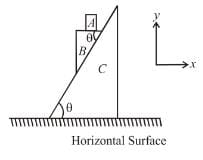Select one or more:

Detailed Solution for Newton's Law Of Motion – MSQ - Question 8

There is no horizontal force on block A, therefore it does not move in x-direction, whereas there is net downwards force (mg – N) is acting on it, making its acceleration along negative y-direction.
Block B moves downwards as well as in negative x-direction. Downward acceleration of A and B will be equal due to constrain, thus w.r.t. BA moves in positive x-direction.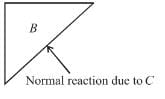Due to the component of normal exerted by C on B, it moves in negative x-direction.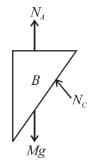The force acting vertically downwards on block B are mg and NA (normal reaction due to block A). Hence the component of net force on block B along the inclined surface of B is greater than mg sin θ. Therefore the acceleration of ‘B’ relative to ground directed along the inclined surface of ‘C’ is greater than g sin θ.

The correct answers are: Acceleration of ‘A’ relative to ground is in negative y-direction, Acceleration of ‘A’ relative to B is in positive x-direction, The horizontal acceleration of ‘B’ relative to ground is in negative x-direction., The acceleration of ‘B’ relative to ground directed along the inclined surface of ‘C’ is greater than gsinθ

*Multiple options can be correct
Newton's Law Of Motion – MSQ - Question 9

A block of mass m is placed on a wedge. The wedge can be accelerated in four manners marked as (1), (2), (3) and (4) as shown. If the normal reactions in situation (1), (2), (3) and (4) are N1N2N3 and N4 respectively and acceleration with which the block slides on the wedge in situations are b1b2b3 and b4 respectively then :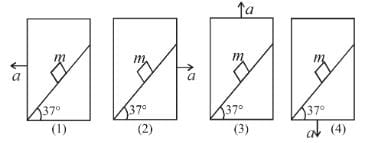Select one or more:

Detailed Solution for Newton's Law Of Motion – MSQ - Question 9

1.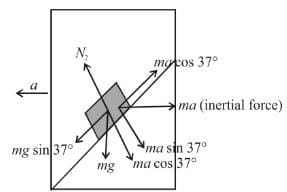Balancing forces perpendicular to incline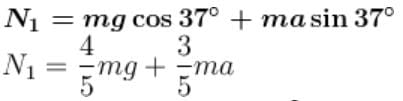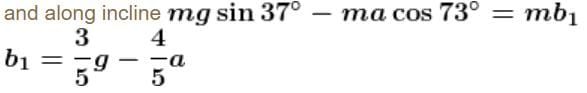2.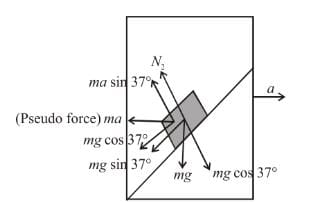Similarly for this case get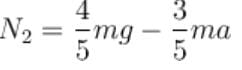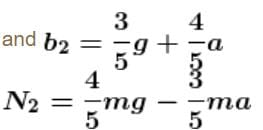3.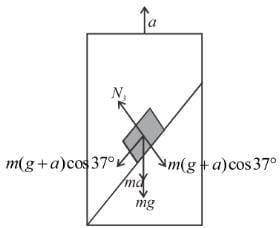Similarly for this case get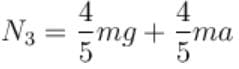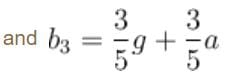4.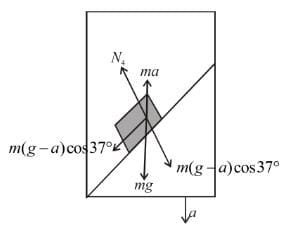Similarly for this case get,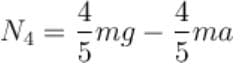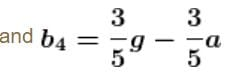The correct answers are: N3 > N1 > N2 > N4 , b2 > b3 > b4 > b1

*Multiple options can be correct
Newton's Law Of Motion – MSQ - Question 10

A cylinder of mass M and radius R is resting on two corner edges A and B as shown in the figure. The normal reaction at the edges A and B are : (Neglect friction)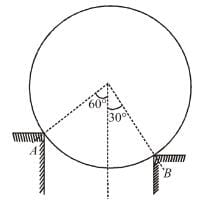Select one or more:

Detailed Solution for Newton's Law Of Motion – MSQ - Question 10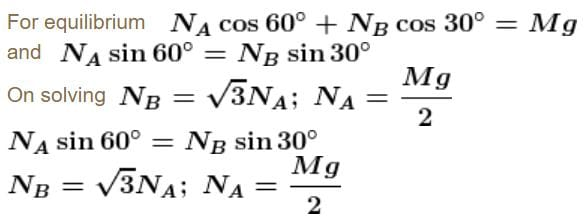The correct answers are: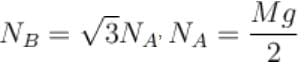## Topic wise Tests for IIT JAM Physics

217 tests
 Use Code STAYHOME200 and get INR 200 additional OFF Use Coupon Code
Information about Newton's Law Of Motion – MSQ Page
In this test you can find the Exam questions for Newton's Law Of Motion – MSQ solved & explained in the simplest way possible. Besides giving Questions and answers for Newton's Law Of Motion – MSQ, EduRev gives you an ample number of Online tests for practice

217 tests